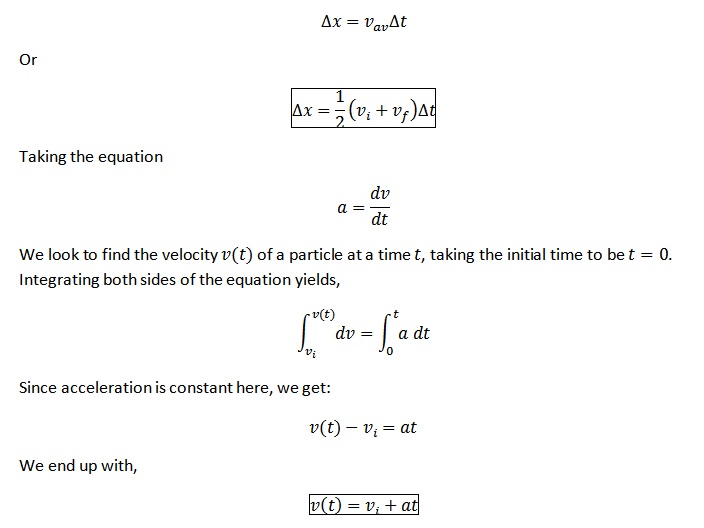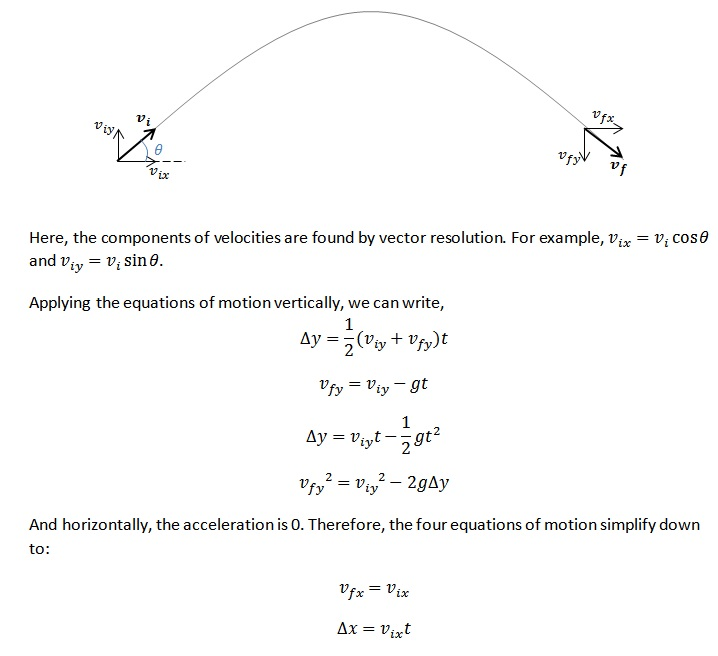# What is Kinematics in Physics

## Definition of Kinematics

Kinematics, in physics, is the study of motion of particles or systems of particles, without taking into consideration the masses of the particles or the forces that cause them to move.

Study of quantities such as displacement, velocity, and acceleration fall under the purview of kinematics in physics.

## What is Displacement

Displacement measures the difference between a particle’s initial and final position. If the position vector of the particle’s initial position,$\vec{r_{i}}$ , is$\vec{r_{i}} = \left (r_{ix}, r_{iy}, r_{iz}\right )$ and the position vector of the particle’s final position,$\vec{r_{f}}$, is$\vec{r_{f}} = \left (r_{fx}, r_{fy}, r_{fz}\right )$, then the displacement$\Delta\vec{r}$ of the particle is given by:$\Delta\vec{r} = \vec{r_{f}} - \vec{r_{i}} = \left (r_{fx} - r_{ix}, r_{fy} - r_{iy}, r_{fz} - r_{iz}\right )$.

## What is Velocity

Velocity is the rate of change of position with respect to time. It is defined as:$\vec{v} = \lim_{\Delta t\rightarrow 0} \frac{\Delta x}{\Delta t} = \frac{\mathrm{d} \vec{r}}{\mathrm{d} t}$.

## What is Acceleration

Acceleration is the rate of change of velocity with respect to time. It is defined as:$\vec{a} = \lim_{\Delta t\rightarrow 0} \frac{\Delta v}{\Delta t} = \frac{\mathrm{d} \vec{v}}{\mathrm{d} t}$.

## What is One-dimensional Kinematics

One-dimensional kinematics is the kinematics of particles moving along a line, i.e., in one spatial dimension.

Under one-dimensional kinematics in physics, we consider a particle moving along a straight line. We can derive equations of motion for the special case where the acceleration is constant. In deriving these equations of motion, we will assume that the particle is moving only in a straight line along the$x$-axis.

If acceleration is uniform, then, over a given period of time$\Delta t$, the average velocity$v_{av}$ is given by$\frac{1}{2} (v_{f} + v_{i})$, where$v_{i}$  is the velocity of the particle at the beginning of the time period and$v_{f}$ is the velocity of the particle at the end of the time period. In this case, the total displacement,$\Delta x$, is simply the product of the average velocity and time:## What is Two-dimensional Kinematics

Two-dimensional kinematics is concerned with particles moving in a plane, i.e., in two spatial dimensions.

With two-dimensional kinematics in physics, in order to analyse two-dimensional motion, we resolve all vector components into two directions which are perpendicular to each other (for instance, the$x$– and$y$-axes on the Cartesian plane, or “vertical” and “horizontal” directions). Motion along one of these directions is then independent of the motion along the other. Consequently, equations of motion can be applied to each of these directions separately.

For example, consider a cannonball shot off from the ground at an angle$\theta$ to the horizontal. In the$y$-direction, the cannonball experiences a constant acceleration of$a_{y} = g=$ -9.81 m s-2. Horizontally, the acceleration is 0, assuming air resistance is negligible.## How to Solve Projectile Motion Problems

References

Kirkby, L. A. (2011). Physics A Student Companion. Scion Publishing.

Whittaker, E. T. (1904). A Treatise on the Analytical Dynamics of Particles & Rigid Bodies. Cambridge University Press.